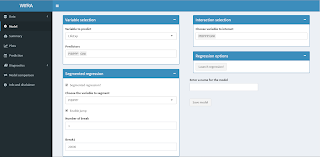# Blog Archives

## A web interface for regression analysis: Walkthrough

June 18, 2016
ByAfter the quick overview, here is a quick walkthrough to some categorical analysis.Open the app: Here1. Import the data:Here are some homemade data, done with the following R code: set.seed(3467)x=1:400+rnorm(400,0,1)y1=x*2.5+40+rnorm(400,0,50)y2=x*4.5+80+rnorm(400,0,50)group=rep(c('G1','G2'),each=400)x=c(x,x)y=c(y1,y2)DF=data.frame(x=x,y=y,group=group)write.csv(DF,'DF.csv')Click on import data, select your data and set rownames to first column. You should then get a quick overview of the data:

## A web interface for regression analysis: Overview

June 18, 2016
ByA Web interface for regression analysis (aka WIfRA) 1.What is it ?Firstly, it was supposed to be a project to learn Shiny and quickly turn into a real project. I wanted to bring data visualisation, regression analysis technique and data engineering to everybody and for no-cost. Basically, this is a point and click UI to do some advanced linear regression...